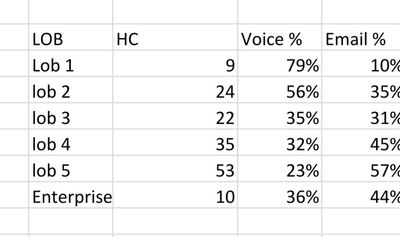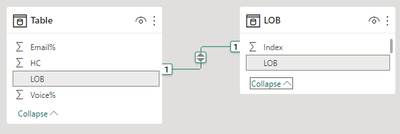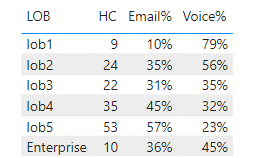cancel
Showing results for
Did you mean:Regular Visitor

## Matrix total calculation

Hi everyone,

I've been struggling to find a way to calculate the total of a matrix.

I'm trying to divide the sum total of the column by 15.

Is there a way to do that?

Like in excel, we can just sum up the table and divide it by any numbers, but I can't do it in power bi1 ACCEPTED SOLUTIONCommunity Support

Hi @mavdom18 ,

In Power BI, the Total row can't be customized. If you want to get the expected result, you need to create a new table contains value "Enterprise" and define its value. Here's my solution:

1.Create a new table. Sort LOB by Index column.Create relationship between the two tables with LOB column.2.Create three measures.

``````HC =
IF (
SUM ( 'Table'[HC] ) <> BLANK (),
SUM ( 'Table'[HC] ),
SUMX ( ALL ( 'Table' ), 'Table'[HC] ) / 15
)
``````
``````Email% =
IF (
MAX ( 'Table'[Email%] ) <> BLANK (),
MAX ( 'Table'[Email%] ),
AVERAGEX ( ALL ( 'Table' ), 'Table'[Email%] )
)
``````
``````Voice% =
IF (
MAX ( 'Table'[Voice%] ) <> BLANK (),
MAX ( 'Table'[Voice%] ),
AVERAGEX ( ALL ( 'Table' ), 'Table'[Voice%] )
)
``````

Get the result:I attach my sample below for your reference.

Best Regards,
Community Support Team _ kalyj

If this post helps, then please consider Accept it as the solution to help the other members find it more quickly.

3 REPLIES 3Community Support

Hi @mavdom18 ,

In Power BI, the Total row can't be customized. If you want to get the expected result, you need to create a new table contains value "Enterprise" and define its value. Here's my solution:

1.Create a new table. Sort LOB by Index column.Create relationship between the two tables with LOB column.2.Create three measures.

``````HC =
IF (
SUM ( 'Table'[HC] ) <> BLANK (),
SUM ( 'Table'[HC] ),
SUMX ( ALL ( 'Table' ), 'Table'[HC] ) / 15
)
``````
``````Email% =
IF (
MAX ( 'Table'[Email%] ) <> BLANK (),
MAX ( 'Table'[Email%] ),
AVERAGEX ( ALL ( 'Table' ), 'Table'[Email%] )
)
``````
``````Voice% =
IF (
MAX ( 'Table'[Voice%] ) <> BLANK (),
MAX ( 'Table'[Voice%] ),
AVERAGEX ( ALL ( 'Table' ), 'Table'[Voice%] )
)
``````

Get the result:I attach my sample below for your reference.

Best Regards,
Community Support Team _ kalyj

If this post helps, then please consider Accept it as the solution to help the other members find it more quickly.Super User

@mavdom18 , Not very clear

example is

divide( calculate(sum(Table[HC]), allselected() ), 15)Regular Visitor

Apologies, I just realized that the image is confusing. To give more context, enterprise is my grand total. Instead of it being just the sum of all the lob combined, I want it to be divided by 15. However, the problem is that it's not as easy as doing it in excel where I can just edit the total formula to be divided by 15.

• What I wanted to do is to make a calculation in my matrix total so that it sums up all the lob and then divide it by 15.Announcements#### Exclusive opportunity for Women!

Join us for a free, hands-on Microsoft workshop led by women trainers for women where you will learn how to build a Dashboard in a Day!#### Power Platform Conference-Power BI and Fabric Sessions

Join us Oct 1 - 6 in Las Vegas for the Microsoft Power Platform Conference.Top Solution Authors
Top Kudoed Authors
Users online (1,744)